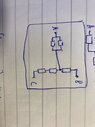# Black box which contains 5x 1ohm resistors

• Engineering
• altruan23

#### altruan23

Homework Statement
I solved it but im not sure if correct, resistance circuit problem.
Relevant Equations
r1*r2/r1+r2 --> 2 parallel resistors.
r1+r2 --> seriel resistors.
You are given a black box with three terminals, as shown below. The box is known to
contain five 1-ohm resistors. Using an ohm-meter, you measure the resistance between the terminals to be the following:
A - B: 1.5 ohms
B - C: 3 ohms
A - C: 2.5 ohms
Determine the configuration of the five resistors inside the box.
So on the left side is the Terminal A, on the right side is B and below is C.

#### Attachments

•image0(7).jpeg
36.1 KB · Views: 92
Last edited:

•